# 5 Essential Indicators Used in Technical Analysis

Share00:00 / 00:00

TL;DR

Indicators are the weapons of choice for battle-tested technical analysts. Each player will choose tools that best fit their unique playstyle to then learn how to master their craft. Some like to look at market momentum, while others want to filter out market noise or measure volatility.

But which are the best technical indicators? Well, every trader will tell you something different. What one analyst will swear is the ultimate indicator another will dismiss completely. However, there are some very popular ones, like the ones we’ve listed below (RSI, MA, MACD, StochRSI, and BB).

Interested to know what they are and how to use them? Read on.

Contents

## Introduction

Traders use technical indicators to gain additional insight into the price action of an asset. These indicators make it easier to identify patterns and spot buy or sell signals in the current market environment. There are many different types of indicators, and they are widely used by day traders, swing traders, and sometimes even longer-term investors. Some professional analysts and advanced traders even create their own indicators. In this article, we’ll provide a brief description of some of the most popular technical analysis indicators that can be useful in any trader’s market analysis toolkit.

## 1. Relative Strength Index (RSI)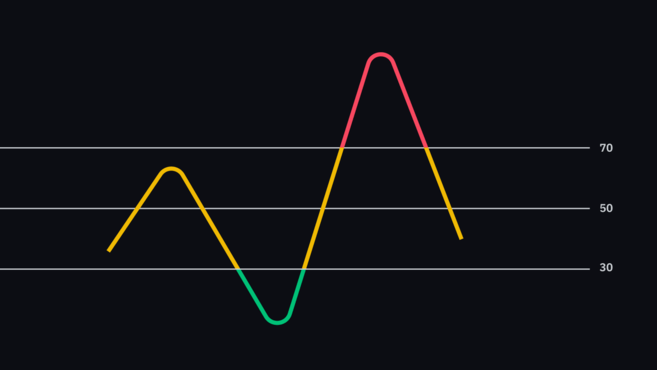The RSI is a momentum indicator that shows whether an asset is overbought or oversold. It does this by measuring the magnitude of recent price changes (the standard setting is the previous 14 periods – so 14 days, 14 hours, etc.). The data is then displayed as an oscillator that can have a value between 0 and 100.

Since the RSI is a momentum indicator, it shows the rate (momentum) at which the price is changing. This means that if momentum is increasing while the price is rising, the uptrend is strong, and more and more buyers are stepping in. In contrast, if momentum is decreasing while the price is rising, it may show that sellers soon might take control over the market.

A traditional interpretation of the RSI is that when it’s over 70, the asset is overbought, and when it’s under 30, it is oversold. As such, extreme values may indicate an impending trend reversal or pullback. Even so, it might be best not to think about these values as direct buy or sell signals. As with many other technical analysis (TA) techniques, the RSI may provide false or misleading signals, so it’s always useful to consider other factors before entering a trade.

Eager to learn more? Check out our article on the Relative Strength Index (RSI).

## 2. Moving Average (MA)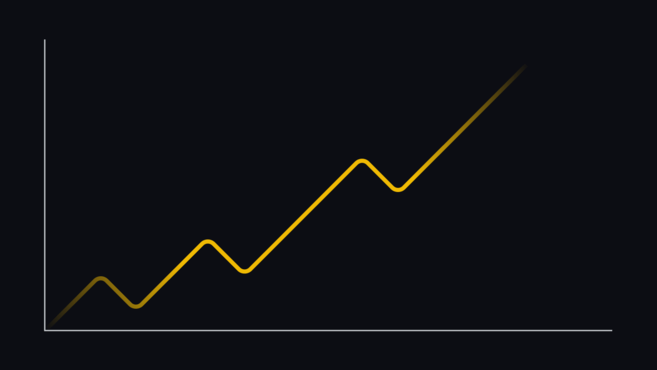A moving average smooths out price action by filtering out market noise and highlighting the direction of the trend. As it’s based on past price data, it’s a lagging indicator.

The two most commonly used moving averages are the simple moving average (SMA or MA), and the exponential moving average (EMA). The SMA is plotted by taking price data from the defined period and producing an average. For example, the 10-day SMA is plotted by calculating the average price over the last 10 days. The EMA, on the other hand, is calculated in a way that gives more weight to recent price data. This makes it more reactive to recent price action.

As mentioned, the moving average is a lagging indicator. The longer the period, the greater the lag. As such, the 200-day SMA will react slower to recent price action than the 50-day SMA.

Traders often use the relationship of the price to specific moving averages to gauge the current market trend. For example, if the price stays above the 200-day SMA for a prolonged period, the asset may be considered to be in a bull market by many traders.

Traders may also use moving average crossovers as buy or sell signals. For example, if the 100-day SMA crosses below the 200-day SMA, it may be considered a sell signal. But what exactly does this cross mean? It indicates that the average price over the last 100 days is now below that of the last 200 days. The idea behind selling here is that short-term price movements are no longer following the uptrend, so the trend may be reversing.

## 3. Moving Average Convergence Divergence (MACD)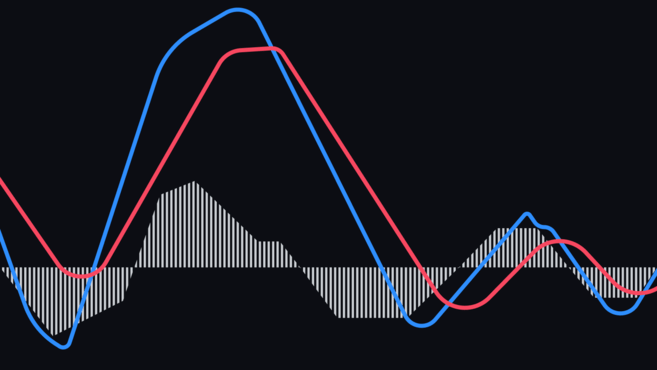The MACD is used to determine the momentum of an asset by showing the relationship between two moving averages. It’s made up of two lines – the MACD line and the signal line. The MACD line is calculated by subtracting the 26 EMA from the 12 EMA. This is then plotted over the MACD line’s 9 EMA – the signal line. Many charting tools also often incorporate a histogram, which shows the distance between the MACD line and the signal line.

By looking for divergences between the MACD and the price action, traders might gain insight into the strength of the current trend. For example, if the price is making a higher high, while the MACD is making a lower high, the market may be reversing soon. What is the MACD telling us in this case? That price is increasing while momentum is decreasing, so there is a higher probability of a pullback or reversal occuring.

Traders may also use this indicator to look for crossovers between the MACD line and its signal line. For example, if the MACD line crosses above the signal line, that may suggest a buy signal. Conversely, if the MACD line crosses below the signal line, that may indicate a sell signal.

The MACD is often used in combination with the RSI, as they both measure momentum, but by different factors. The assumption is that together they may give a more complete technical outlook on the market.

Looking to get started with cryptocurrency? Buy Bitcoin on Binance!

## 4. Stochastic RSI (StochRSI)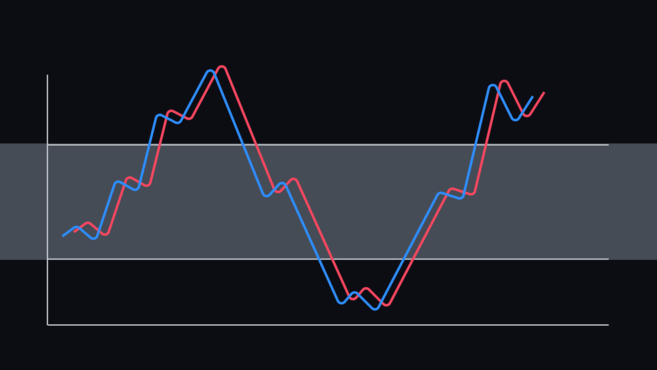The Stochastic RSI is a momentum oscillator used to determine whether an asset is overbought or oversold. As the name suggests, it’s a derivative of the RSI, as it’s generated from RSI values instead of price data. It’s created by applying a formula called the Stochastic oscillator formula to the ordinary RSI values. Typically, the Stochastic RSI values range between 0 and 1 (or 0 and 100).

Due to its greater speed and sensitivity, the StochRSI can generate a lot of trading signals that can be tricky to interpret. Generally, it tends to be the most useful when near the upper or lower extremes of its range.

A StochRSI reading above 0.8 is usually considered overbought, while a value below 0.2 may be considered oversold. A value of 0 means that the RSI is at its lowest value in the measured period (the default setting is typically 14). Conversely, a value of 1 represents that the RSI is at its highest value in the measured period.

Similarly to how the RSI should be used, an overbought or oversold StochRSI value doesn’t mean that the price will surely reverse. In the case of the StochRSI, it simply indicates that the RSI values (which StochRSI values are derived from) are near the extremes of their recent readings. It’s also important to keep in mind that the StochRSI is more sensitive than the RSI indicator, so it tends to generate more false or misleading signals.

Eager to learn more? Check out our article on the Stochastic RSI.

## 5. Bollinger Bands (BB)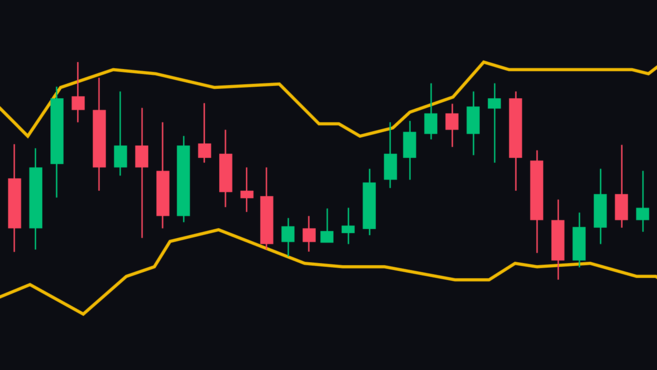Bollinger Bands measure the volatility of the market, as well as overbought and oversold conditions. They are made up of three lines - an SMA (the middle band), and an upper and lower band. The settings may vary, but typically the upper and lower bands are two standard deviations away from the middle band. As volatility increases and decreases, the distance between the bands increases and decreases as well.

Generally, the closer the price is to the upper band, the closer to overbought conditions the charted asset may be. Conversely, the closer the price is to the lower band, the closer to oversold conditions it may be. For the most part, price will stay within the bands, but on rare occasions, it may break above or below them. While this event may not be a trading signal in itself, it can act as an indication of extreme market conditions.

Another important concept of BBs is called the squeeze. It refers to a period of low volatility, where all bands come very close to each other. This may be used as an indication of potential future volatility. Conversely, if the bands are very far from each other, a period of decreased volatility may follow.